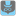• # Hidden Word, Iterating Vertically?

## Question related to missionThe Hidden Word

Alright, so my code creates a list of every line within text, checks those lines horizontally for word and returns the coordinates of word if it's there. Last thing, if word isn't in the list horizontally, how might I iterate over the list vertically, check for word that way and return the coordinates.

```def checkio(text, word):
coordinates = []
word = word.lower()
text = text.lower()
text = text.replace(" ", "")
text = text.split("\n")
for item in text:
if word in item:
row_start = text.index(item)
coordinates.append(row_start)
col_start = item.index(word)
coordinates.append(col_start)
coordinates.append(row_start)
col_end = item.index(word) #Add length of word to
coordinates.append(col_end)
coordinates += 1
coordinates += 1
coordinates += 1
coordinates += len(word)
return coordinates
```